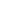# PiThe value of the mathematical constant pi. The number π is a mathematical constant that is the ratio of a circle’s circumference to its diameter.